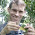## Wednesday, March 20, 2013

### Conditional scaled likelihoods in ace & on not using them for ancestral state reconstruction

A not very well appreciated attribute of ace(...,type="discrete") is that the scaled likelihoods returned in the matrix \$lik.anc are the scaled conditional likelihoods from the "pruning" algorithm of Felsenstein (1981), and not the joint or marginal reconstructions - which we should generally prefer for ancestral state estimation. This was pointed out to me a couple of years ago by Rich Fitzjohn. It is not stated in the documentation for ace that this is the case, but it is implied in Paradis' new book - if a little obtusely. Specifically, on p. 254 Paradis says "if the option CI=TRUE is used, then the likelihood of each ancestral state is returned for each node in a matrix called lik.anc. They are computed with a formula similar to (5.9)....". If we go to formula (5.9) on p. 147, we see that it is the formula for the conditional likelihood of the subtree from the pruning algorithm.

One trivial way to "demonstrate" (and I use this term loosely) that this is the case is by simulating & then analyzing trees with branches of zero length. This is because nodes separated by a branch of zero length will have identical joint/marginal reconstructions - but they may have different conditional reconstructions from the pruning algorithm because these scaled likelihoods consider only the subtree descended from the node.

Here's a super quick demo of what I mean:

> require(phytools)
> tree<-pbtree(n=30,scale=2) # simulate tree
> # set some branches to zero
> tree\$edge.length[tree\$edge.length<0.1]<-0
> # now add some length to terminal branches so that
> # the tree is ultrametric
> plot(tree,no.margin=TRUE,edge.width=2)

OK, good. This tree has some internal branches of zero length. Now let's simulate on the tree & compute the likelihoods with ace:
> # simulate data
> Q<-matrix(c(-2,1,1,1,-2,1,1,1,-2),3,3)
> rownames(Q)<-colnames(Q)<-letters[1:3]
> x<-sim.history(tree,Q)\$states
> # use ace & plot scaled likelihoods
> XX<-ace(x,tree,type="discrete")
> piesXX<-XX\$lik.anc
> rownames(piesXX)<-1:tree\$Nnode+length(tree\$tip)
> tips<-sapply(letters[1:3],"==",x)*1
> plot(tree,no.margin=TRUE,show.tip.label=FALSE, edge.width=2)
> nodelabels(pie=piesXX,piecol=setNames(c("blue","red", "green"),colnames(pies)),cex=0.7)
> tiplabels(pie=tips,piecol=setNames(c("blue","red", "green"),colnames(pies)),cex=0.7)
Obviously, from this plot we see that nodes separated with a branch of zero length (apparently polytomies in this graph) have different conditional scaled likelihoods, as we'd expect. Here, we can visualize why by plotting the tree without edge lengths:
> nodelabels(pie=piesXX,piecol=setNames(c("blue","red", "green"),colnames(pies)),cex=0.7)
> tiplabels(pie=tips,piecol=setNames(c("blue","red", "green"),colnames(pies)),cex=0.7)

An alternative is to use stochastic mapping, which should (asymptotically, as the number of simulations is increased) converge to the joint [correction, marginal] likelihood reconstructions (I believe). Here's how to do it using phytools. Note that the latest version of phytools is recommended as there is a bug in make.simmap in most earlier versions:

> # check phytools package version
> packageVersion("phytools")
 ‘0.2.30’
> # do it using make.simmap
> mtrees<-make.simmap(tree,x,nsim=200,model="ER")
make.simmap is sampling character histories conditioned on the transition matrix
Q =
a          b          c
a -1.3631646  0.6815823  0.6815823
b  0.6815823 -1.3631646  0.6815823
c  0.6815823  0.6815823 -1.3631646
(estimated using likelihood);
and state frequencies (used to sample the root node following Bollback 2006)
pi =
a         b         c
0.3333333 0.3333333 0.3333333
> # function to compute the states at each node
> foo<-function(x){
y<-sapply(x\$maps,function(x) names(x))
names(y)<-x\$edge[,1]
y<-y[as.character(length(x\$tip)+1:x\$Nnode)]
return(y)
}
> AA<-sapply(mtrees,foo)
> piesAA<-t(apply(AA,1,function(x,levels,Nsim) summary(factor(x,levels))/Nsim,levels=letters[1:3], Nsim=length(mtrees)))
> plot(tree,no.margin=TRUE,show.tip.label=FALSE, edge.width=2)
> nodelabels(pie=piesAA,piecol=setNames(c("blue","red", "green"),colnames(pies)),cex=0.7)
> tiplabels(pie=tips,piecol=setNames(c("blue","red", "green"),colnames(pies)),cex=0.7)
Much better. Now nodes separated by branches of zero length have the same probabilities - which they must as no evolution could occur on said branches.

Let me note that this is not intended to be a criticism of ace(...,type="discrete") - I just think that it may not be doing what many people think it is doing and this should be of concern.

1.Oh, and I believe that joint reconstruction can be performed using Rich Fitzjohn's package diversitree. - Liam

2.Marginal & joint reconstruction is evidently possible in diversitree.

I should also say that after thinking about this some more, the method above based on stochastic mapping gives the marginal probabilities for the ancestral states based on a joint sampling procedure. (This is also stated about SIMMAP, here.) These seem like they will be very close to the marginal probabilities, but perhaps not exactly the same.

3.Hi Liam,

you can also compute the ancestral states in phangorn:

library(phangorn)
X = phyDat(as.matrix(x), type="USER", levels=c("a", "b", "c"))
fit = pml(tree, X)
# you may want to optimize the overall rate
fit = optim.pml(fit, optEdge=FALSE, optRate=TRUE)
fit\$rate
anc.ml = ancestral.pml(fit)
plotAnc(tree, anc.ml, 1)

Slightly different angle as normally you optimize with optim.pml the tree and keep the rates fixed/scaled.

Cheers,
Klaus

PS: I always found it a bit problematic that functions like sim.history seem not to scale the rate matrix. For DNA there is a kind of convention to set the transition G->T to one as you can easily compare different rate matrices. Internally Q is scaled differently see e.g. formula (13.14) p. 205 in Felsensteins "Inferring phylogenies". So it is still possible that users mess around with the edge length of the tree.

1.Thanks Klaus. This works quite well. I suppose I should have realized that this could be done with phangorn!

Yes, in tree inference, the branch lengths are not fixed so we need to fix (or scale) Q.

In comparative inference, the branch lengths are fixed (because the tree is already estimated) and may be in units of time, so we do not need to scale Q. When we estimate Q this puts it on the scale of the branch lengths of the input tree.

Thanks for posting this! Liam

Note: due to the very large amount of spam, all comments are now automatically submitted for moderation.